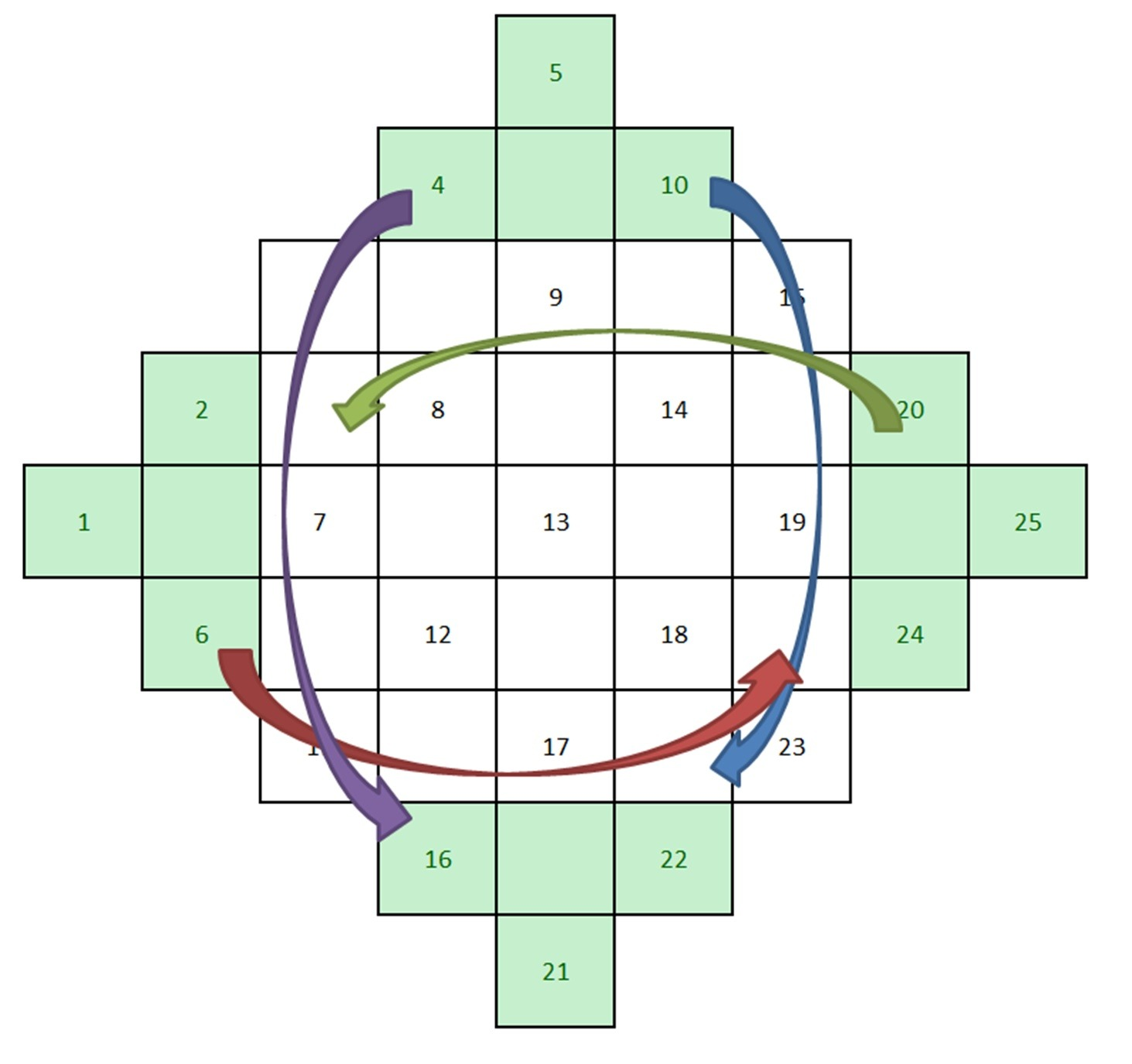# Category: Interesting math

During the 14th century Europe was devastated by the plague. Since middle ages medicine was extremely basic, they had no power over this terrible disease. People were terrified because they believed that Black Death was punishment from God.Image credit: Wikimedia Commons user Nicolas Poussin

They resorted to less verified sources and massively became superstitious. Here came the idea that mathematics can help prevent diseases and keep the devil at bay. Few good mathematicians created so called magic square – a square table of order n containing numbers from 1 to n2 so that the sum of numbers in each row, column and both diagonals is the same. Soon enough, magic squares were everywhere.Image credit to Eve Andersson

## How to construct the magic squares of any order?

Order of the magic square represents the number of its rows or columns.

Construction of magic squares is divided into two different procedures – one where its order is odd and one where it’s even.

First we’ll show the construction of magic squares with odd order.

Start with an empty square and draw a grid in it. For example we’ll make a magic square whose order is 5.Divide every side on 5 parts and draw parallels to make a grid with 25 squares.

Now on every side of this square we’ll construct a “triangle” of 4 squares.Now, starting from the top left diagonal, draw numbers from 1 to 25 in every other diagonal (including the added “triangles”).If the order is $n$, your last diagonal should end with n2. Now we should simply fill in the gaps. You take the numbers from those triangles and translate them vertically or horizontally to the furthest free place. If the number is placed on the top triangle you’ll move it down, if it’s on the left you’ll move it right and so on.And the final result is:Now we’ll show construction of magic squares of even order.

It is impossible to construct a magic square whose order is equal to 2. We’ll show how to construct a magic square whose order is equal to 4.

Draw a grid 4 x 4 and color them like this:Starting from the bottom right corner, write numbers from 1 to $n_2$.The numbers in the white cells are here to stay. But the numbers in the pink cells must be rearranged.

Now erase the number in the pink cells and start the counting again from the top right number corner.Now, the numbers in the white cells will remain as they were, and pink cells will take the numbers from the counting. This is the final result:Of course, you can test it out. The sum of all rows should be equal to the sum of all columns and the sum of diagonal numbers.

The number Pi has been known for almost 4000 years. Pi is one of the most fascinating numbers.

Imagine: if you write down an alphabet and you give each letter a certain number, in some part of pi your whole future can be written. This is because pi is infinite and there is no sequence of numbers that is repeating. Pi is ratio of the circumference of a circle and its diameter. The ancient Babylonians gave very rough approximation to pi- they estimated it to 3. The first real calculations were done by Archimedes of Syracuse.Image credit: Wikimedia Commons user John Reid

Pi is ratio of the circumference of a circle and its diameter. The ancient Babylonians gave very rough approximation to pi- they estimated it to 3. The first real calculations were done by Archimedes of Syracuse. He showed that pi is one number between $3 \frac{1}{4}$ and $3 \frac{10}{71}$. He approximated the area of a circle based on the area of a regular polygon inscribed within the circle and the area of a regular polygon within which the circle was circumscribed.Through all history, people found pi interesting. They wanted to know pi to more decimals, but couldn’t. Then the great Indian mathematical genius – Srinivasa Ramanujan started observing pi. He discovered remarkable formula for computing pi:But it still all remained as estimation.

Later on, using the computer Alexander J. Yee and Shiger Kondo claimed to have calculated number pi to 5 trillion places. The main computation took 90 days.Now we use the standard approximation to $\pi – 3.14$. But what if we want to remember pi to more than two decimals? We’d want to write it down as a fraction. For this we’ll use continued fraction.

Remember that the continued fractions come in form:Let’s first take that $\pi = 3.14159$.

First number will be the floor of the starting number:

$\mid a_0 \mid = \mid 3.14159 \mid = 3$

Now we have to calculate the first help variable $b_1$:

$3.14159 = a_0 + \frac{1}{b_1} \rightarrow b1 = 7.0625$

Using the help variable we can calculate $a_1$:

$a_1 = \mid b_1 \mid = \mid 7.0625 \mid = 7 \rightarrow a_1 = 7$

Now you simply continue the process.

$b_1 = a_1 + \frac{1}{b_2} \rightarrow b_2 = 15.96424$

$a_2 = \mid b_2 \mid = \mid 15.9642 \mid = 15\rightarrow a_2 = 15$

$b_2 = a_2 + \frac{1}{b_3} \rightarrow b_3 = 1.037$

$a_3 = \mid b3 \mid = \mid 1.037 \mid = 1\rightarrow a_3 = 1$

This calculation leads us to our fraction approximation of pi:

$\pi \approx [3, 7, 15, 1] = \frac{3 + 1}{7 + \frac{1}{15 + \frac{1}{2}}}$

Now you’ll simplify this fraction and get that:

$\pi \approx \frac{335}{113} = 3.141592$

Also, don’t forget to celebrate March 14 (3/14), the international Pi Day!